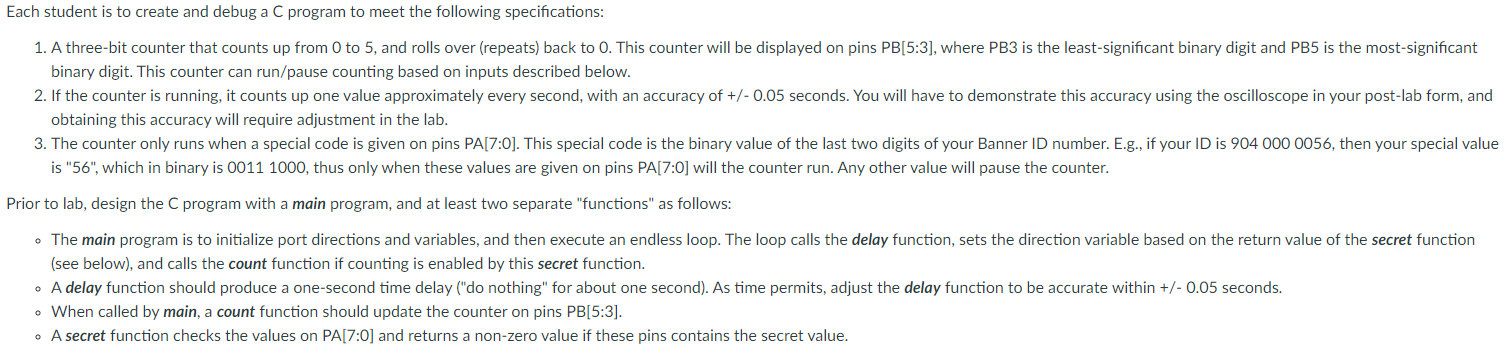Home / Expert Answers / Computer Science / include-34-stm32l4xx-h-34-microcontroller-information-define-global-variables-static-int-va-pa298

# (Solved): #include "stm32l4xx.h" /* Microcontroller information */ /* Define global variables */ static int va ...

#include "stm32l4xx.h" /* Microcontroller information */

/* Define global variables */
static int value1, value2; /*counter values */

unsigned static char run = 0; //controls if the counters are counting
unsigned volatile char up = 0; //controls which direction the first one is counting
unsigned static char led1, led2 = 0; //led1 == red led - represents state of "run" variable. led2 == ylw led - represents state of "up" variable.

void PinSetup () {
/* Configure PA1, PA2 as input pin to read the switches */
RCC->AHB2ENR |= 0x01; /* Enable GPIOA clock (bit 0) */
GPIOA->MODER &= ~(0x0000003C); /* General purpose input mode */
/* Configure PB[5:3] as output pins to drive DIO LEDs */
GPIOA->MODER &= ~(0x03FFFC00); /* Clear PB[5:3] mode bits */
GPIOA->MODER |=    (0x01555400); /* General purpose output mode for PB[5:3]*/
/* Configure PB4,PB3 as output pins to drive LEDs */
RCC->AHB2ENR |= 0x02; /* Enable GPIOB clock (bit 1) */
GPIOB->MODER &= ~(0x000003C0); /* Clear PB4-PB3 mode bits */
GPIOB->MODER |=    (0x00000140); /* General purpose output mode*/

}

/*----------------------------------------------------------*/
/* Delay function - do nothing for about 0.05 second */
/*----------------------------------------------------------*/
void delay () {
int volatile i,j,n;
for (i=0; i<20; i++) { //outer loop
for (j=0; j<20000; j++) { //inner loop
n = j; //dummy operation for single-step test
} //do nothing
}
}

/*----------------------------------------------------------*/
/* Count function - increment/decrement the counters */
/*----------------------------------------------------------*/
void count() {
if(up==0){
value1++;
if(value1==6){
value1=0;
}
}else{
value1--;
if(value1==-1){
value1=5;
}
}
value2++;
if(value2==6){
value2=0;
}
GPIOA->ODR = (value1 << 5) //Write value1 to PB[5:3]
}

/*------------------------------------------------*/
/* Main program */
/*------------------------------------------------*/
int main(void) {
PinSetup(); //Configure GPIO pins

/* Endless loop */
while (1) {
delay();
run = GPIOA->IDR &= 0x0002; //PA1 = switch, which is also the "run" value.
up = GPIOA->IDR &= 0x0004; //PA2 = switch, which is also the "UP/DOWN" value.
if(run != 0){ //A COMMON MISTAKE! "Set" is not "1".
count(); //Never being called? Look at the assembly code, as see if "run" is never being loaded. If not, you need to declare it differently.
}
} /* repeat forever */
}how to fix the program and my special value is 85

binary digit. This counter can run/pause counting based on inputs described below. obtaining this accuracy will require adjustment in the lab. is "56", which in binary is 00111000 , thus only when these values are given on pins PA[7:0] will the counter run. Any other value will pause the counter. Prior to lab, design the C program with a main program, and at least two separate "functions" as follows: (see below), and calls the count function if counting is enabled by this secret function. - When called by main, a count function should update the counter on pins PB[5:3]. - A secret function checks the values on $$\mathrm{PA}[7: 0]$$ and returns a non-zero value if these pins contains the secret value.

We have an Answer from Expert